GFG App
Open AppBrowser
Continue

# Introduction to Max-Heap – Data Structure and Algorithm Tutorials

A Max-Heap is defined as a type of Heap Data Structure in which each internal node is greater than or equal to its children.

The heap data structure is a type of binary tree that is commonly used in computer science for various purposes, including sorting, searching, and organizing data.Introduction to Max-Heap Data Structure

Purpose and Use Cases of Max-Heap:

• Priority Queue: One of the primary uses of the heap data structure is for implementing priority queues.
• Heap Sort: The heap data structure is also used in sorting algorithms.
• Memory Management: The heap data structure is also used in memory management. When a program needs to allocate memory dynamically, it uses the heap data structure to keep track of the available memory.
• Graph Algorithms: The heap data structure is used in various graph algorithms. For example, Dijkstra’s shortest path algorithm uses a heap data structure to keep track of the vertices with the shortest path from the source vertex.

## Max-Heap Data structure in Different languages:

### 1. Max-Heap in C++

A max heap can be implemented using the priority_queue container from the Standard Template Library (STL). The priority_queue container is a type of container adapter that provides a way to store elements in a queue-like data structure in which each element has a priority associated with it.

`Syntax: priority_queue<int>maxH;`

### 2. Max-Heap in Java

In Java, a max heap can be implemented using the PriorityQueue class from java.util package. The PriorityQueue class is a priority queue that provides a way to store elements in a queue-like data structure in which each element has a priority associated with it.

`Syntax: PriorityQueue maxHeap= new PriorityQueue<>(Comparator.reverseOrder());`

### 3. Max-Heap in Python

In Python, a max heap can be implemented using the heapq module, which provides functions for implementing heaps. Specifically, the heapq module provides a way to create and manipulate heap data structures.

```Syntax:  heap = []
heapify(heap)```

### 4. Max-Heap in C#

In C#, a max heap can be implemented using the PriorityQueue<T> class from the System.Collections.Generic namespace. The PriorityQueue<T> class is a priority queue that provides a way to store elements in a queue-like data structure in which each element has a priority associated with it.

`Syntax: var maxHeap = new PriorityQueue<int>((a, b) => b - a);`

### 5. Max-Heap in JavaScript

A max heap is a binary tree where every node has a value greater than or equal to its children. In JavaScript, you can implement a max heap using an array, where the first element represents the root node, and the children of a node at index i are located at indices 2i+1 and 2i+2.

`Syntax: const miaxHeap = new MaxHeap();`

## Internal Implementation of Max-Heap Data Structure:

A Min heap is typically represented as an array

• The root element will be at Arr
• For any ith node Arr[i].
• left child is stored at index 2i+1
• Right child is stored at index 2i+2
• Parent is stored at index floor((i-1)/2)

The Internal Implementation of the Max-Heap require 3 major steps:

1. Insertion: To insert a new element into the heap, it is added to the end of the array and then “bubbled up” until it satisfies the heap property.
2. Deletion: To delete the maximum element (the root of the heap), the last element in the array is swapped with the root, and the new root is “bubbled down” until it satisfies the heap property.
3. Heapify: A heapify operation can be used to create a max heap from an unsorted array.

## Operations on Max-heap Data Structure and their Implementation:

Here are some common operations that can be performed on a Heap Data Structure data structure,

## 1. Insertion in Max-Heap Data Structure:

Elements can be inserted to the heap following a similar approach as discussed above for deletion. The idea is to:

• First increase the heap size by 1, so that it can store the new element.
• Insert the new element at the end of the Heap.
• This newly inserted element may distort the properties of Heap for its parents. So, in order to keep the properties of Heap, heapify this newly inserted element following a bottom-up approach.

Illustration:

Suppose the Heap is a Max-Heap as: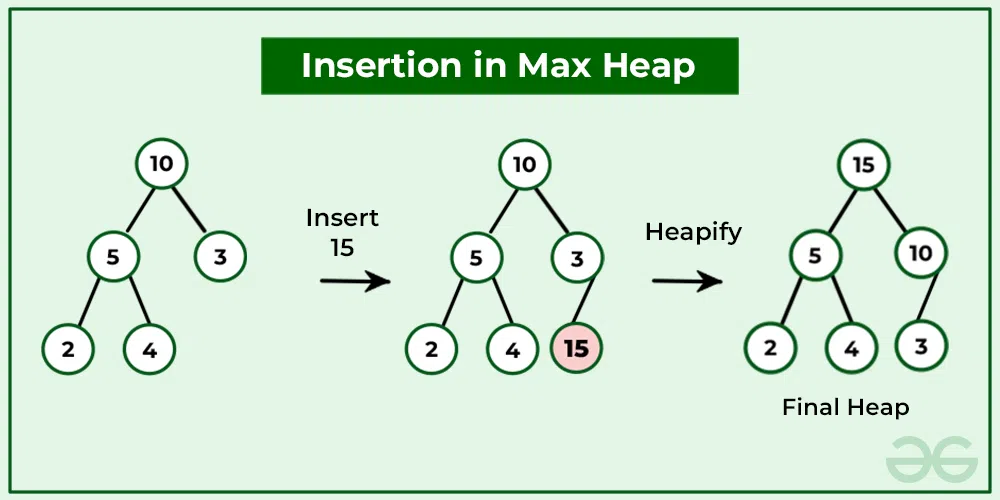Insertion in Max-Heap

## C++

 `// C++ program to insert new element to Heap`   `#include ` `using` `namespace` `std;`   `#define MAX 1000 // Max size of Heap`   `// Function to heapify ith node in a Heap` `// of size n following a Bottom-up approach` `void` `heapify(``int` `arr[], ``int` `n, ``int` `i)` `{` `    ``// Find parent` `    ``int` `parent = (i - 1) / 2;`   `    ``if` `(arr[parent] > 0) {` `        ``// For Max-Heap` `        ``// If current node is greater than its parent` `        ``// Swap both of them and call heapify again` `        ``// for the parent` `        ``if` `(arr[i] > arr[parent]) {` `            ``swap(arr[i], arr[parent]);`   `            ``// Recursively heapify the parent node` `            ``heapify(arr, n, parent);` `        ``}` `    ``}` `}`   `// Function to insert a new node to the Heap` `void` `insertNode(``int` `arr[], ``int``& n, ``int` `Key)` `{` `    ``// Increase the size of Heap by 1` `    ``n = n + 1;`   `    ``// Insert the element at end of Heap` `    ``arr[n - 1] = Key;`   `    ``// Heapify the new node following a` `    ``// Bottom-up approach` `    ``heapify(arr, n, n - 1);` `}`   `// A utility function to print array of size n` `void` `printArray(``int` `arr[], ``int` `n)` `{` `    ``for` `(``int` `i = 0; i < n; ++i)` `        ``cout << arr[i] << ``" "``;`   `    ``cout << ``"\n"``;` `}`   `// Driver Code` `int` `main()` `{` `    ``// Array representation of Max-Heap` `    ``// 10` `    ``// / \` `    ``// 5 3` `    ``// / \` `    ``// 2 4` `    ``int` `arr[MAX] = { 10, 5, 3, 2, 4 };`   `    ``int` `n = 5;`   `    ``int` `key = 15;`   `    ``insertNode(arr, n, key);`   `    ``printArray(arr, n);` `    ``// Final Heap will be:` `    ``// 15` `    ``// / \` `    ``// 5     10` `    ``// / \ /` `    ``// 2 4 3` `    ``return` `0;` `}`

## Java

 `// Java program for implementing insertion in Heaps` `public` `class` `insertionHeap {`   `    ``// Function to heapify ith node in a Heap` `    ``// of size n following a Bottom-up approach` `    ``static` `void` `heapify(``int``[] arr, ``int` `n, ``int` `i)` `    ``{` `        ``// Find parent` `        ``int` `parent = (i - ``1``) / ``2``;` `    `  `        ``if` `(arr[parent] > ``0``) {` `            ``// For Max-Heap` `            ``// If current node is greater than its parent` `            ``// Swap both of them and call heapify again` `            ``// for the parent` `            ``if` `(arr[i] > arr[parent]) {` `                `  `                ``// swap arr[i] and arr[parent]` `                ``int` `temp = arr[i];` `                ``arr[i] = arr[parent];` `                ``arr[parent] = temp;` `            `  `                ``// Recursively heapify the parent node` `                ``heapify(arr, n, parent);` `            ``}` `        ``}` `    ``}`   `    ``// Function to insert a new node to the heap.` `    ``static` `int` `insertNode(``int``[] arr, ``int` `n, ``int` `Key)` `    ``{` `        ``// Increase the size of Heap by 1` `        ``n = n + ``1``;` `    `  `        ``// Insert the element at end of Heap` `        ``arr[n - ``1``] = Key;` `    `  `        ``// Heapify the new node following a` `        ``// Bottom-up approach` `        ``heapify(arr, n, n - ``1``);` `        `  `        ``// return new size of Heap` `        ``return` `n;` `    ``}`   `    ``/* A utility function to print array of size n */` `    ``static` `void` `printArray(``int``[] arr, ``int` `n)` `    ``{` `        ``for` `(``int` `i = ``0``; i < n; ++i)` `            ``System.out.println(arr[i] + ``" "``);`   `        ``System.out.println();` `    ``}`   `    ``// Driver Code` `    ``public` `static` `void` `main(String args[])` `    ``{` `        ``// Array representation of Max-Heap` `        ``// 10` `        ``// / \` `        ``// 5 3` `        ``// / \` `        ``// 2 4` `        `  `        ``// maximum size of the array` `        ``int` `MAX = ``1000``;` `        ``int``[] arr = ``new` `int``[MAX];` `        `  `        ``// initializing some values` `        ``arr[``0``] = ``10``;` `        ``arr[``1``] = ``5``;` `        ``arr[``2``] = ``3``;` `        ``arr[``3``] = ``2``;` `        ``arr[``4``] = ``4``;` `        `  `        ``// Current size of the array` `        ``int` `n = ``5``;`   `        ``// the element to be inserted` `        ``int` `Key = ``15``;` `        `  `        ``// The function inserts the new element to the heap and` `        ``// returns the new size of the array` `        ``n = insertNode(arr, n, Key);`   `        ``printArray(arr, n);` `        ``// Final Heap will be:` `        ``// 15` `        ``// / \` `        ``// 5     10` `        ``// / \ /` `        ``// 2 4 3` `    ``}` `}`   `// The code is contributed by Gautam goel`

## Python3

 `# program to insert new element to Heap`   `# Function to heapify ith node in a Heap` `# of size n following a Bottom-up approach`     `def` `heapify(arr, n, i):` `    ``parent ``=` `int``(((i``-``1``)``/``2``))` `    ``# For Max-Heap` `    ``# If current node is greater than its parent` `    ``# Swap both of them and call heapify again` `    ``# for the parent` `    ``if` `arr[parent] > ``0``:` `        ``if` `arr[i] > arr[parent]:` `            ``arr[i], arr[parent] ``=` `arr[parent], arr[i]` `            ``# Recursively heapify the parent node` `            ``heapify(arr, n, parent)` `# Function to insert a new node to the Heap`     `def` `insertNode(arr, key):` `    ``global` `n` `    ``# Increase the size of Heap by 1` `    ``n ``+``=` `1` `    ``# Insert the element at end of Heap` `    ``arr.append(key)` `    ``# Heapify the new node following a` `    ``# Bottom-up approach` `    ``heapify(arr, n, n``-``1``)` `# A utility function to print array of size n`     `def` `printArr(arr, n):` `    ``for` `i ``in` `range``(n):` `        ``print``(arr[i], end``=``" "``)`     `# Driver Code` `# Array representation of Max-Heap` `'''` `        ``10` `    ``/ \` `    ``5 3` `    ``/ \` `    ``2 4` `'''` `arr ``=` `[``10``, ``5``, ``3``, ``2``, ``4``, ``1``, ``7``]` `n ``=` `7` `key ``=` `15` `insertNode(arr, key)` `printArr(arr, n)` `# Final Heap will be:` `'''` `    ``15` `    ``/ \` `5     10` `/ \ /` `2 4 3`   `Code is written by Rajat Kumar....` `'''`

## C#

 `// C# program for implementing insertion in Heaps`   `using` `System;`   `public` `class` `insertionHeap {`   `    ``// Function to heapify ith node in a Heap of size n following a Bottom-up approach` `    ``static` `void` `heapify(``int``[] arr, ``int` `n, ``int` `i) {` `        ``// Find parent` `        ``int` `parent = (i - 1) / 2;`   `        ``if` `(arr[parent] > 0) {` `            ``// For Max-Heap` `            ``// If current node is greater than its parent` `            ``// Swap both of them and call heapify again` `            ``// for the parent` `            ``if` `(arr[i] > arr[parent]) {`   `                ``// swap arr[i] and arr[parent]` `                ``int` `temp = arr[i];` `                ``arr[i] = arr[parent];` `                ``arr[parent] = temp;`   `                ``// Recursively heapify the parent node` `                ``heapify(arr, n, parent);` `            ``}` `        ``}` `    ``}`   `    ``// Function to insert a new node to the heap.` `    ``static` `int` `insertNode(``int``[] arr, ``int` `n, ``int` `Key) {` `        ``// Increase the size of Heap by 1` `        ``n = n + 1;`   `        ``// Insert the element at end of Heap` `        ``arr[n - 1] = Key;`   `        ``// Heapify the new node following a` `        ``// Bottom-up approach` `        ``heapify(arr, n, n - 1);`   `        ``// return new size of Heap` `        ``return` `n;` `    ``}`   `    ``/* A utility function to print array of size n */` `    ``static` `void` `printArray(``int``[] arr, ``int` `n) {` `        ``for` `(``int` `i = 0; i < n; ++i)` `            ``Console.WriteLine(arr[i] + ``" "``);`   `        ``Console.WriteLine(``""``);` `    ``}`   `    ``public` `static` `void` `Main(``string``[] args) {` `        ``// Array representation of Max-Heap` `        ``//     10` `        ``// / \` `        ``// 5 3` `        ``// / \` `        ``// 2 4`   `        ``// maximum size of the array` `        ``int` `MAX = 1000;` `        ``int``[] arr = ``new` `int``[MAX];`   `        ``// initializing some values` `        ``arr = 10;` `        ``arr = 5;` `        ``arr = 3;` `        ``arr = 2;` `        ``arr = 4;`   `        ``// Current size of the array` `        ``int` `n = 5;`   `        ``// the element to be inserted` `        ``int` `Key = 15;`   `        ``// The function inserts the new element to the heap and` `        ``// returns the new size of the array` `        ``n = insertNode(arr, n, Key);`   `        ``printArray(arr, n);` `        ``// Final Heap will be:` `        ``//     15` `        ``// / \` `        ``// 5     10` `        ``// / \ /` `        ``// 2 4 3` `    ``}` `}`   `// This code is contributed by ajaymakvana.`

## Javascript

 `// Javascript program for implement insertion in Heaps`   `// To heapify a subtree rooted with node i which is` `// an index in arr[].Nn is size of heap`   `let MAX = 1000;`   `// Function to heapify ith node in a Heap of size n following a Bottom-up approach` `function` `heapify(arr, n, i)` `{` `    ``// Find parent` `    ``let parent = Math.floor((i-1)/2);`   `    ``if` `(arr[parent] >= 0) {` `        ``// For Max-Heap` `        ``// If current node is greater than its parent` `        ``// Swap both of them and call heapify again` `        ``// for the parent` `        ``if` `(arr[i] > arr[parent]) {` `            ``let temp = arr[i];` `            ``arr[i] = arr[parent];` `            ``arr[parent] = temp;`   `            ``// Recursively heapify the parent node` `            ``heapify(arr, n, parent);` `        ``}` `    ``}` `}`   `// Function to insert a new node to the Heap` `function` `insertNode(arr, n, Key)` `{` `    ``// Increase the size of Heap by 1` `    ``n = n + 1;`   `    ``// Insert the element at end of Heap` `    ``arr[n - 1] = Key;`   `    ``// Heapify the new node following a` `    ``// Bottom-up approach` `    ``heapify(arr, n, n - 1);` `    `  `    ``return` `n;` `}`   `/* A utility function to print array of size N */` `function` `printArray(arr, n)` `{` `    ``for` `(let i = 0; i < n; ++i)` `        ``console.log(arr[i] + ``" "``);`   `    ``console.log(``"
"``);` `}`   `let arr = [ 10, 5, 3, 2, 4 ];`   `let n = arr.length;`   `let key = 15;`   `n = insertNode(arr, n, key);`   `printArray(arr, n);`   `// This code is contributed by ajaymakvana`

Output

`15 5 10 2 4 3 `

Time Complexity:  O(log(n)) (where n is no of elements in the heap)
Auxiliary Space: O(n)

## 2. Deletion in Max-Heap Data Structure:

Deleting an element at any intermediary position in the heap can be costly, so we can simply replace the element to be deleted with the last element and delete the last element of the Heap.

• Replace the root or element to be deleted with the last element.
• Delete the last element from the Heap.
• Since the last element is now placed at the position of the root node. So, it may not follow the heap property. Therefore, heapify the last node placed at the position of the root.

Illustration:

Suppose the Heap is a Max-Heap as: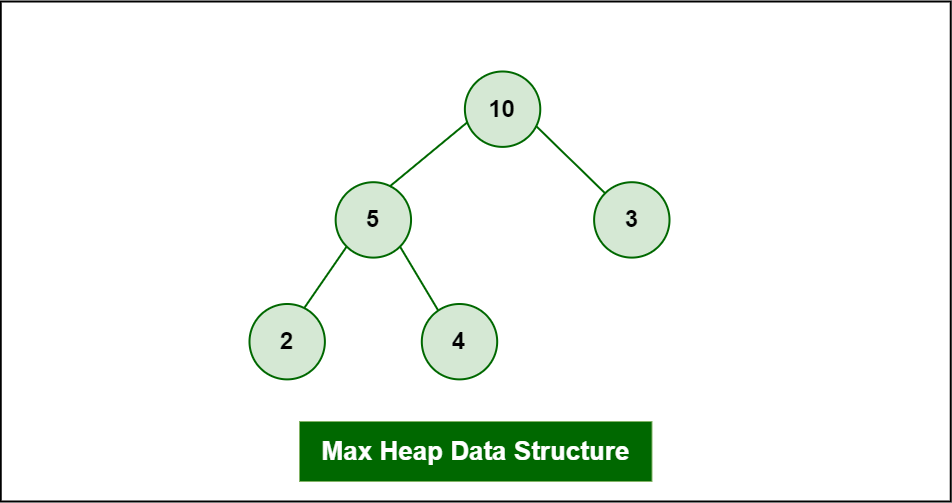Deletion in Max-Heap

The element to be deleted is root, i.e. 10.

Process:

The last element is 4.

Step 1: Replace the last element with root, and delete it.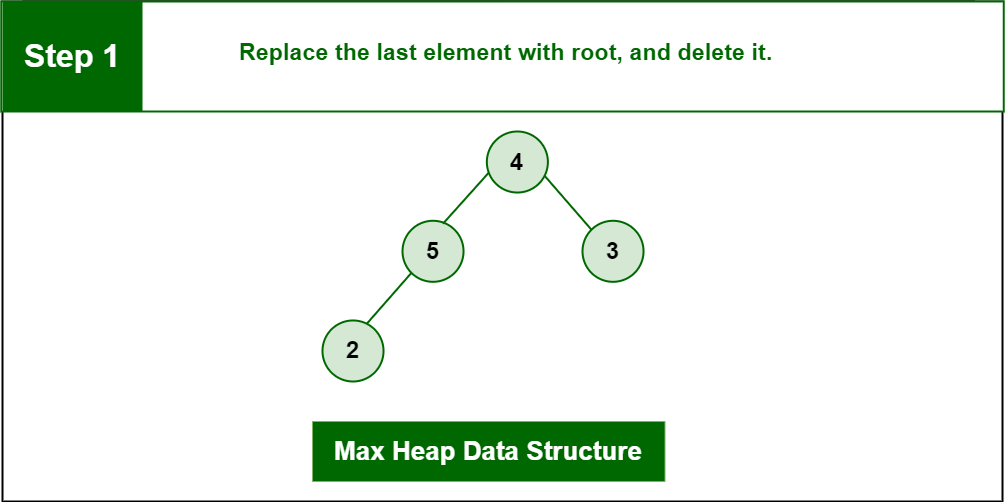Deletion in Max-Heap

Step 2: Heapify root.

Final Heap: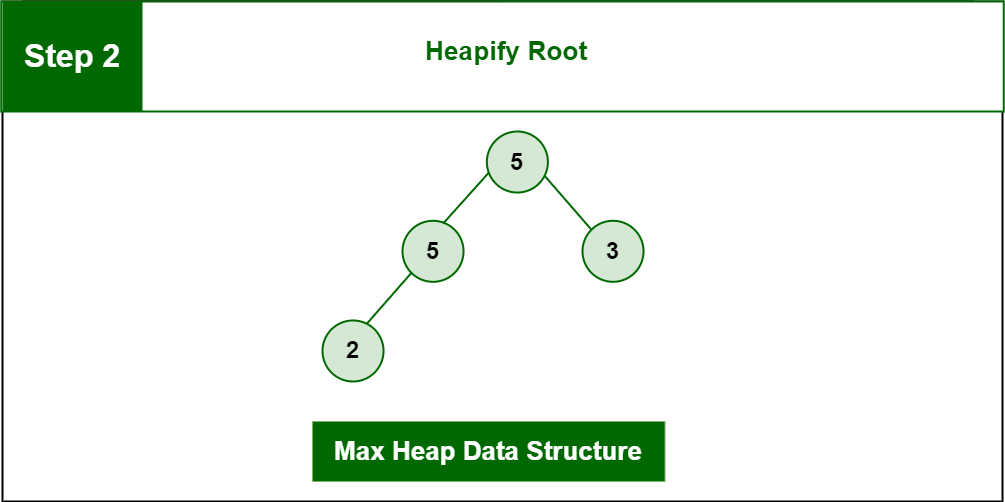Deletion in Max-Heap

## C++

 `// C++ program for implement deletion in Heaps`   `#include `   `using` `namespace` `std;`   `// To heapify a subtree rooted with node i which is` `// an index of arr[] and n is the size of heap` `void` `heapify(``int` `arr[], ``int` `n, ``int` `i)` `{` `    ``int` `largest = i; ``// Initialize largest as root` `    ``int` `l = 2 * i + 1; ``// left = 2*i + 1` `    ``int` `r = 2 * i + 2; ``// right = 2*i + 2`   `    ``// If left child is larger than root` `    ``if` `(l < n && arr[l] > arr[largest])` `        ``largest = l;`   `    ``// If right child is larger than largest so far` `    ``if` `(r < n && arr[r] > arr[largest])` `        ``largest = r;`   `    ``// If largest is not root` `    ``if` `(largest != i) {` `        ``swap(arr[i], arr[largest]);`   `        ``// Recursively heapify the affected sub-tree` `        ``heapify(arr, n, largest);` `    ``}` `}`   `// Function to delete the root from Heap` `void` `deleteRoot(``int` `arr[], ``int``& n)` `{` `    ``// Get the last element` `    ``int` `lastElement = arr[n - 1];`   `    ``// Replace root with last element` `    ``arr = lastElement;`   `    ``// Decrease size of heap by 1` `    ``n = n - 1;`   `    ``// heapify the root node` `    ``heapify(arr, n, 0);` `}`   `/* A utility function to print array of size n */` `void` `printArray(``int` `arr[], ``int` `n)` `{` `    ``for` `(``int` `i = 0; i < n; ++i)` `        ``cout << arr[i] << ``" "``;` `    ``cout << ``"\n"``;` `}`   `// Driver Code` `int` `main()` `{` `    ``// Array representation of Max-Heap` `    ``//     10` `    ``// / \` `    ``// 5 3` `    ``// / \` `    ``// 2 4` `    ``int` `arr[] = { 10, 5, 3, 2, 4 };`   `    ``int` `n = ``sizeof``(arr) / ``sizeof``(arr);`   `    ``deleteRoot(arr, n);`   `    ``printArray(arr, n);`   `    ``return` `0;` `}`

## Java

 `// Java program for implement deletion in Heaps` `public` `class` `deletionHeap {`   `    ``// To heapify a subtree rooted with node i which is` `    ``// an index in arr[].Nn is size of heap` `    ``static` `void` `heapify(``int` `arr[], ``int` `n, ``int` `i)` `    ``{` `        ``int` `largest = i; ``// Initialize largest as root` `        ``int` `l = ``2` `* i + ``1``; ``// left = 2*i + 1` `        ``int` `r = ``2` `* i + ``2``; ``// right = 2*i + 2`   `        ``// If left child is larger than root` `        ``if` `(l < n && arr[l] > arr[largest])` `            ``largest = l;`   `        ``// If right child is larger than largest so far` `        ``if` `(r < n && arr[r] > arr[largest])` `            ``largest = r;`   `        ``// If largest is not root` `        ``if` `(largest != i) {` `            ``int` `swap = arr[i];` `            ``arr[i] = arr[largest];` `            ``arr[largest] = swap;`   `            ``// Recursively heapify the affected sub-tree` `            ``heapify(arr, n, largest);` `        ``}` `    ``}`   `    ``// Function to delete the root from Heap` `    ``static` `int` `deleteRoot(``int` `arr[], ``int` `n)` `    ``{` `        ``// Get the last element` `        ``int` `lastElement = arr[n - ``1``];`   `        ``// Replace root with first element` `        ``arr[``0``] = lastElement;`   `        ``// Decrease size of heap by 1` `        ``n = n - ``1``;`   `        ``// heapify the root node` `        ``heapify(arr, n, ``0``);`   `        ``// return new size of Heap` `        ``return` `n;` `    ``}`   `    ``/* A utility function to print array of size N */` `    ``static` `void` `printArray(``int` `arr[], ``int` `n)` `    ``{` `        ``for` `(``int` `i = ``0``; i < n; ++i)` `            ``System.out.print(arr[i] + ``" "``);`   `        ``System.out.println();` `    ``}`   `    ``// Driver Code` `    ``public` `static` `void` `main(String args[])` `    ``{` `        ``// Array representation of Max-Heap` `        ``// 10` `        ``// / \` `        ``// 5 3` `        ``// / \` `        ``// 2 4` `        ``int` `arr[] = { ``10``, ``5``, ``3``, ``2``, ``4` `};`   `        ``int` `n = arr.length;`   `        ``n = deleteRoot(arr, n);`   `        ``printArray(arr, n);` `    ``}` `}`

## Python3

 `# Python 3 program for implement deletion in Heaps`   `# To heapify a subtree rooted with node i which is` `# an index of arr[] and n is the size of heap` `def` `heapify(arr, n, i):`   `    ``largest ``=` `i ``#Initialize largest as root` `    ``l ``=` `2` `*` `i ``+` `1` `# left = 2*i + 1` `    ``r ``=` `2` `*` `i ``+` `2` `# right = 2*i + 2`   `    ``#If left child is larger than root` `    ``if` `(l < n ``and` `arr[l] > arr[largest]):` `        ``largest ``=` `l`   `    ``#If right child is larger than largest so far` `    ``if` `(r < n ``and` `arr[r] > arr[largest]):` `        ``largest ``=` `r`   `    ``# If largest is not root` `    ``if` `(largest !``=` `i):` `        ``arr[i],arr[largest]``=``arr[largest],arr[i]`   `        ``#Recursively heapify the affected sub-tree` `        ``heapify(arr, n, largest)`   `#Function to delete the root from Heap` `def` `deleteRoot(arr):` `    ``global` `n`   `    ``# Get the last element` `    ``lastElement ``=` `arr[n ``-` `1``]`   `    ``# Replace root with last element` `    ``arr[``0``] ``=` `lastElement`   `    ``# Decrease size of heap by 1` `    ``n ``=` `n ``-` `1`   `    ``# heapify the root node` `    ``heapify(arr, n, ``0``)`   `# A utility function to print array of size n` `def` `printArray(arr, n):`   `    ``for` `i ``in` `range``(n):` `        ``print``(arr[i],end``=``" "``)` `    ``print``()`   `# Driver Code` `if` `__name__ ``=``=` `'__main__'``:`   `    ``# Array representation of Max-Heap` `    ``#     10` `    ``#     / \` `    ``# 5 3` `    ``# / \` `    ``# 2 4` `    ``arr ``=` `[ ``10``, ``5``, ``3``, ``2``, ``4` `]`   `    ``n ``=` `len``(arr)`   `    ``deleteRoot(arr)`   `    ``printArray(arr, n)` `    `  `    ``# This code is contributed by Rajat Kumar.`

## C#

 `// C# program for implement deletion in Heaps` `using` `System;`   `public` `class` `deletionHeap` `{`   `    ``// To heapify a subtree rooted with node i which is` `    ``// an index in arr[].Nn is size of heap` `    ``static` `void` `heapify(``int` `[]arr, ``int` `n, ``int` `i)` `    ``{` `        ``int` `largest = i; ``// Initialize largest as root` `        ``int` `l = 2 * i + 1; ``// left = 2*i + 1` `        ``int` `r = 2 * i + 2; ``// right = 2*i + 2`   `        ``// If left child is larger than root` `        ``if` `(l < n && arr[l] > arr[largest])` `            ``largest = l;`   `        ``// If right child is larger than largest so far` `        ``if` `(r < n && arr[r] > arr[largest])` `            ``largest = r;`   `        ``// If largest is not root` `        ``if` `(largest != i)` `        ``{` `            ``int` `swap = arr[i];` `            ``arr[i] = arr[largest];` `            ``arr[largest] = swap;`   `            ``// Recursively heapify the affected sub-tree` `            ``heapify(arr, n, largest);` `        ``}` `    ``}`   `    ``// Function to delete the root from Heap` `    ``static` `int` `deleteRoot(``int` `[]arr, ``int` `n)` `    ``{` `        ``// Get the last element` `        ``int` `lastElement = arr[n - 1];`   `        ``// Replace root with first element` `        ``arr = lastElement;`   `        ``// Decrease size of heap by 1` `        ``n = n - 1;`   `        ``// heapify the root node` `        ``heapify(arr, n, 0);`   `        ``// return new size of Heap` `        ``return` `n;` `    ``}`   `    ``/* A utility function to print array of size N */` `    ``static` `void` `printArray(``int` `[]arr, ``int` `n)` `    ``{` `        ``for` `(``int` `i = 0; i < n; ++i)` `            ``Console.Write(arr[i] + ``" "``);`   `        ``Console.WriteLine();` `    ``}`   `    ``// Driver Code` `    ``public` `static` `void` `Main()` `    ``{` `        ``// Array representation of Max-Heap` `        ``// 10` `        ``// / \` `        ``// 5 3` `        ``// / \` `        ``// 2 4` `        ``int` `[]arr = { 10, 5, 3, 2, 4 };` `        ``int` `n = arr.Length;` `        ``n = deleteRoot(arr, n);` `        ``printArray(arr, n);` `    ``}` `}`   `// This code is contributed by Ryuga`

## Javascript

 ``

Output

`5 4 3 2 `

Time complexity: O(log n) where n is no of elements in the heap
Auxiliary Space: O(n)

## 3. Peek operation on Max-heap Data Structure:

To access the maximum element (i.e., the root of the heap), the value of the root node is returned. The time complexity of peek in a max heap is O(1).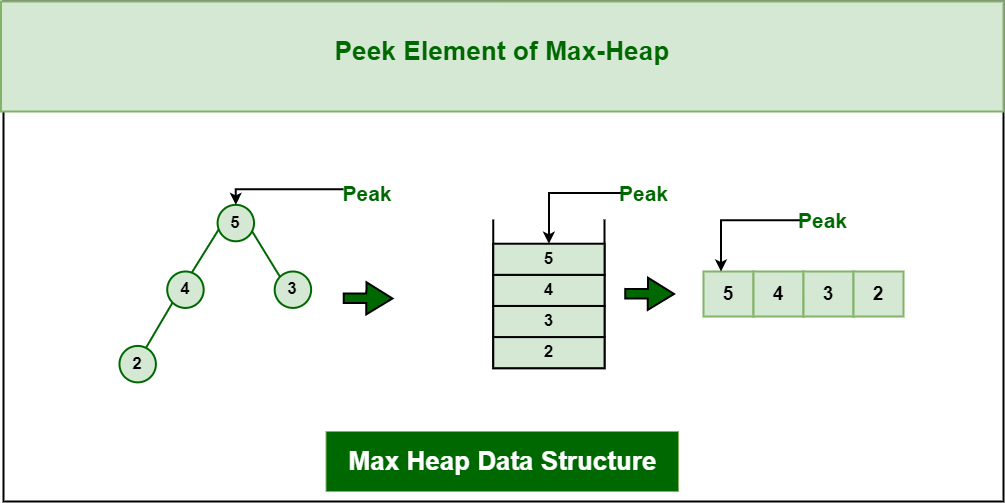Peek Element

## C++

 `#include ` `#include `   `int` `main() {` `    ``// Create a max heap with some elements using a priority_queue` `    ``std::priority_queue<``int``> maxHeap;` `    ``maxHeap.push(9);` `    ``maxHeap.push(8);` `    ``maxHeap.push(7);` `    ``maxHeap.push(6);` `    ``maxHeap.push(5);` `    ``maxHeap.push(4);` `    ``maxHeap.push(3);` `    ``maxHeap.push(2);` `    ``maxHeap.push(1);`   `    ``// Get the peak element (i.e., the largest element)` `    ``int` `peakElement = maxHeap.top();`   `    ``// Print the peak element` `    ``std::cout << ``"Peak element: "` `<< peakElement << std::endl;`   `    ``return` `0;` `}`

## Java

 `import` `java.util.PriorityQueue;`   `public` `class` `GFG {` `    ``public` `static` `void` `main(String[] args) {` `        ``// Create a max heap with some elements using a PriorityQueue` `        ``PriorityQueue maxHeap = ``new` `PriorityQueue<>((a, b) -> b - a);` `        ``maxHeap.add(``9``);` `        ``maxHeap.add(``8``);` `        ``maxHeap.add(``7``);` `        ``maxHeap.add(``6``);` `        ``maxHeap.add(``5``);` `        ``maxHeap.add(``4``);` `        ``maxHeap.add(``3``);` `        ``maxHeap.add(``2``);` `        ``maxHeap.add(``1``);`   `        ``// Get the peak element (i.e., the largest element)` `        ``int` `peakElement = maxHeap.peek();`   `        ``// Print the peak element` `        ``System.out.println(``"Peak element: "` `+ peakElement);` `    ``}` `}`

## Python3

 `import` `heapq`   `# Create a max heap with some elements using a list` `max_heap ``=` `[``1``,``2``,``3``,``4``,``5``,``6``,``7``,``8``,``9``]` `heapq.heapify(max_heap)`   `# Get the peak element (i.e., the largest element)` `peak_element ``=` `heapq.nlargest(``1``, max_heap)[``0``]`   `# Print the peak element` `print``(``"Peak element:"``, peak_element)`

## C#

 `using` `System;` `using` `System.Collections.Generic;`   `public` `class` `GFG {` `    ``public` `static` `void` `Main() {` `        ``// Create a min heap with some elements using a PriorityQueue` `        ``var` `maxHeap = ``new` `PriorityQueue<``int``>();` `        ``maxHeap.Enqueue(9);` `        ``maxHeap.Enqueue(8);` `        ``maxHeap.Enqueue(7);` `        ``maxHeap.Enqueue(6);` `        ``maxHeap.Enqueue(5);` `        ``maxHeap.Enqueue(4);` `        ``maxHeap.Enqueue(3);` `        ``maxHeap.Enqueue(2);` `        ``maxHeap.Enqueue(1);`   `        ``// Get the peak element (i.e., the smallest element)` `        ``int` `peakElement = maxHeap.Peek();`   `        ``// Print the peak element` `        ``Console.WriteLine(``"Peak element: "` `+ peakElement);` `    ``}` `}` `// Define a PriorityQueue class that uses a max heap` `class` `PriorityQueue ``where` `T : IComparable {` `    ``private` `List heap;`   `    ``public` `PriorityQueue() {` `        ``this``.heap = ``new` `List();` `    ``}`   `    ``public` `int` `Count {` `        ``get` `{ ``return` `this``.heap.Count; }` `    ``}`   `    ``public` `void` `Enqueue(T item) {` `        ``this``.heap.Add(item);` `        ``this``.BubbleUp(``this``.heap.Count - 1);` `    ``}`   `    ``public` `T Dequeue() {` `        ``T item = ``this``.heap;` `        ``int` `lastIndex = ``this``.heap.Count - 1;` `        ``this``.heap = ``this``.heap[lastIndex];` `        ``this``.heap.RemoveAt(lastIndex);` `        ``this``.BubbleDown(0);` `        ``return` `item;` `    ``}`   `    ``public` `T Peek() {` `        ``return` `this``.heap;` `    ``}`   `    ``private` `void` `BubbleUp(``int` `index) {` `        ``while` `(index > 0) {` `            ``int` `parentIndex = (index - 1) / 2;` `            ``if` `(``this``.heap[parentIndex].CompareTo(``this``.heap[index]) >= 0) {` `                ``break``;` `            ``}` `            ``Swap(parentIndex, index);` `            ``index = parentIndex;` `        ``}` `    ``}`   `    ``private` `void` `BubbleDown(``int` `index) {` `        ``while` `(index < ``this``.heap.Count) {` `            ``int` `leftChildIndex = index * 2 + 1;` `            ``int` `rightChildIndex = index * 2 + 2;` `            ``int` `largestChildIndex = index;` `            ``if` `(leftChildIndex < ``this``.heap.Count && ``this``.heap[leftChildIndex].CompareTo(``this``.heap[largestChildIndex]) > 0) {` `                ``largestChildIndex = leftChildIndex;` `            ``}` `            ``if` `(rightChildIndex < ``this``.heap.Count && ``this``.heap[rightChildIndex].CompareTo(``this``.heap[largestChildIndex]) > 0) {` `                ``largestChildIndex = rightChildIndex;` `            ``}` `            ``if` `(largestChildIndex == index) {` `                ``break``;` `            ``}` `            ``Swap(largestChildIndex, index);` `            ``index = largestChildIndex;` `        ``}` `    ``}`   `    ``private` `void` `Swap(``int` `i, ``int` `j) {` `        ``T temp = ``this``.heap[i];` `        ``this``.heap[i] = ``this``.heap[j];` `        ``this``.heap[j] = temp;` `    ``}` `}`

## Javascript

 `// Define a MaxHeap class that uses an array` `class MaxHeap {` `    ``constructor() {` `        ``this``.heap = [];` `    ``}`   `    ``push(item) {` `        ``this``.heap.push(item);` `        ``this``.bubbleUp(``this``.heap.length - 1);` `    ``}`   `    ``pop() {` `        ``let item = ``this``.heap;` `        ``let lastIndex = ``this``.heap.length - 1;` `        ``this``.heap = ``this``.heap[lastIndex];` `        ``this``.heap.pop();` `        ``this``.bubbleDown(0);` `        ``return` `item;` `    ``}`   `    ``peak() {` `        ``return` `this``.heap;` `    ``}`   `    ``bubbleUp(index) {` `        ``while` `(index > 0) {` `            ``let parentIndex = Math.floor((index - 1) / 2);` `            ``if` `(``this``.heap[parentIndex] >= ``this``.heap[index]) {` `                ``break``;` `            ``}` `            ``this``.swap(parentIndex, index);` `            ``index = parentIndex;` `        ``}` `    ``}`   `    ``bubbleDown(index) {` `        ``while` `(index < ``this``.heap.length) {` `            ``let leftChildIndex = index * 2 + 1;` `            ``let rightChildIndex = index * 2 + 2;` `            ``let largestChildIndex = index;` `            ``if` `(leftChildIndex < ``this``.heap.length && ``this``.heap[leftChildIndex] > ``this``.heap[largestChildIndex]) {` `                ``largestChildIndex = leftChildIndex;` `            ``}` `            ``if` `(rightChildIndex < ``this``.heap.length && ``this``.heap[rightChildIndex] > ``this``.heap[largestChildIndex]) {` `                ``largestChildIndex = rightChildIndex;` `            ``}` `            ``if` `(largestChildIndex === index) {` `                ``break``;` `            ``}` `            ``this``.swap(largestChildIndex, index);` `            ``index = largestChildIndex;` `        ``}` `    ``}`   `    ``swap(i, j) {` `        ``let temp = ``this``.heap[i];` `        ``this``.heap[i] = ``this``.heap[j];` `        ``this``.heap[j] = temp;` `    ``}` `}`   `// Create a max heap with some elements using an array` `let maxHeap = ``new` `MaxHeap();` `maxHeap.push(9);` `maxHeap.push(8);` `maxHeap.push(7);` `maxHeap.push(6);` `maxHeap.push(5);` `maxHeap.push(4);` `maxHeap.push(3);` `maxHeap.push(2);` `maxHeap.push(1);`   `// Get the peak element (i.e., the largest element)` `let peakElement = maxHeap.peak();`   `// Print the peak element` `console.log(``"Peak element: "` `+ peakElement);`

Output

`Peak element: 9`

Time complexity

• In a max heap implemented using an array or a list, the peak element can be accessed in constant time, O(1), as it is always located at the root of the heap.
• In a max heap implemented using a binary tree, the peak element can also be accessed in O(1) time, as it is always located at the root of the tree.

Auxiliary Space: O(n)

## 4. Heapify operation on Max-heap Data Structure: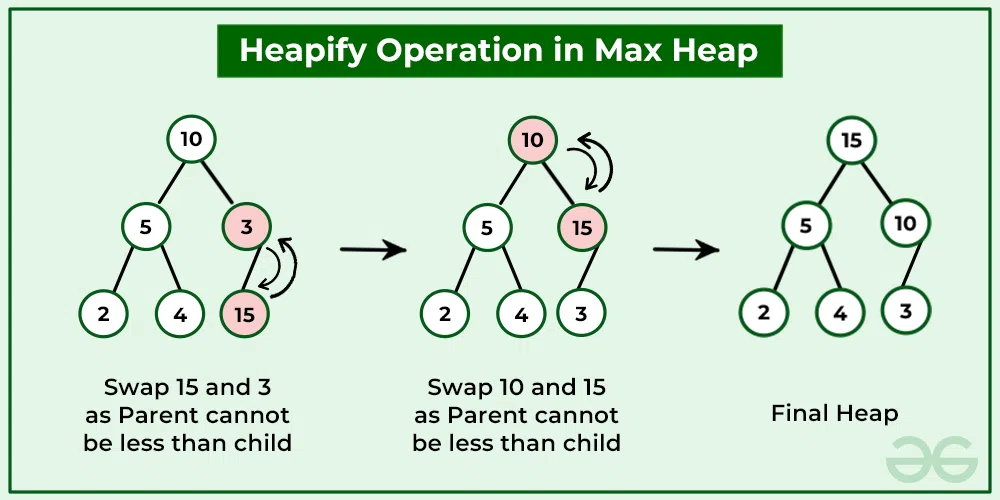Heapify operation

A heapify operation can be used to create a max heap from an unsorted array. This is done by starting at the last non-leaf node and repeatedly performing the “bubble down” operation until all nodes satisfy the heap property. The time complexity of heapify in a max heap is O(n).

## 5. Search operation on Max-heap Data Structure::

To search for an element in the max heap, a linear search can be performed over the array that represents the heap. However, the time complexity of a linear search is O(n), which is not efficient. Therefore, searching is not a commonly used operation in a max heap.

Here’s an example code that shows how to search for an element in a max heap using std::find():

## C++

 `#include ` `#include // for std::priority_queue` `using` `namespace` `std;`   `int` `main() {` `    ``std::priority_queue<``int``> max_heap;` `  ``// example max heap` `  `  `  ``max_heap.push(10);` `  ``max_heap.push(9);` `  ``max_heap.push(8);` `  ``max_heap.push(6);` `  ``max_heap.push(4);`   `    ``int` `element = 6; ``// element to search for` `    ``bool` `found = ``false``;`   `    ``// Copy the max heap to a temporary queue and search for the element` `    ``std::priority_queue<``int``> temp = max_heap;` `    ``while` `(!temp.empty()) {` `        ``if` `(temp.top() == element) {` `            ``found = ``true``;` `            ``break``;` `        ``}` `        ``temp.pop();` `    ``}`   `    ``if` `(found) {` `        ``std::cout << ``"Element found in the max heap."` `<< std::endl;` `    ``} ``else` `{` `        ``std::cout << ``"Element not found in the max heap."` `<< std::endl;` `    ``}`   `    ``return` `0;` `}`

## Java

 `import` `java.util.PriorityQueue;`   `public` `class` `GFG {` `    ``public` `static` `void` `main(String[] args) {` `        ``PriorityQueue maxHeap = ``new` `PriorityQueue<>((a, b) -> b - a);` `        ``maxHeap.add(``3``); ``// insert elements into the priority queue` `        ``maxHeap.offer(``1``);` `        ``maxHeap.offer(``4``);` `        ``maxHeap.offer(``1``);` `        ``maxHeap.offer(``6``);`   `        ``int` `element = ``6``; ``// element to search for` `        ``boolean` `found = ``false``;`   `        ``// Copy the max heap to a temporary queue and search for the element` `        ``PriorityQueue temp = ``new` `PriorityQueue<>(maxHeap);` `        ``while` `(!temp.isEmpty()) {` `            ``if` `(temp.poll() == element) {` `                ``found = ``true``;` `                ``break``;` `            ``}` `        ``}`   `        ``if` `(found) {` `            ``System.out.println(``"Element found in the max heap."``);` `        ``} ``else` `{` `            ``System.out.println(``"Element not found in the max heap."``);` `        ``}` `    ``}` `}`

## Python3

 `import` `heapq`   `max_heap ``=` `[``10``, ``8``, ``7``, ``6``, ``5``, ``3``, ``2``, ``1``] ``# example max heap` `heapq._heapify_max(max_heap)`   `element ``=` `6` `# element to search for` `found ``=` `False`   `# Copy the max heap to a temporary list and search for the element` `temp ``=` `list``(max_heap)` `while` `temp:` `    ``if` `heapq._heappop_max(temp) ``=``=` `element:` `        ``found ``=` `True` `        ``break`   `if` `found:` `    ``print``(``"Element found in the max heap."``)` `else``:` `    ``print``(``"Element not found in the max heap."``)`

## C#

 `using` `System;` `using` `System.Collections.Generic;`   `class` `Program {` `    ``static` `void` `Main(``string``[] args) {` `        ``// Create a max heap with some elements using a PriorityQueue` `        ``PriorityQueue<``int``> maxHeap = ``new` `PriorityQueue<``int``>();` `        ``maxHeap.Enqueue(10);` `        ``maxHeap.Enqueue(9);` `        ``maxHeap.Enqueue(8);` `        ``maxHeap.Enqueue(6);` `        ``maxHeap.Enqueue(4);`   `        ``int` `element = 6; ``// element to search for` `        ``bool` `found = ``false``;`   `        ``// Copy the max heap to a temporary queue and search for the element` `        ``PriorityQueue<``int``> temp = ``new` `PriorityQueue<``int``>(maxHeap);` `        ``while` `(temp.Count > 0) {` `            ``if` `(temp.Peek() == element) {` `                ``found = ``true``;` `                ``break``;` `            ``}` `            ``temp.Dequeue();` `        ``}`   `        ``if` `(found) {` `            ``Console.WriteLine(``"Element found in the max heap."``);` `        ``} ``else` `{` `            ``Console.WriteLine(``"Element not found in the max heap."``);` `        ``}` `    ``}` `}`   `// PriorityQueue class` `class` `PriorityQueue ``where` `T : IComparable {` `    ``private` `List heap = ``new` `List();`   `    ``public` `void` `Enqueue(T item) {` `        ``heap.Add(item);` `        ``int` `child = heap.Count - 1;` `        ``while` `(child > 0) {` `            ``int` `parent = (child - 1) / 2;` `            ``if` `(heap[child].CompareTo(heap[parent]) > 0) {` `                ``T tmp = heap[child];` `                ``heap[child] = heap[parent];` `                ``heap[parent] = tmp;` `                ``child = parent;` `            ``} ``else` `{` `                ``break``;` `            ``}` `        ``}` `    ``}`   `    ``public` `T Dequeue() {` `        ``int` `last = heap.Count - 1;` `        ``T frontItem = heap;` `        ``heap = heap[last];` `        ``heap.RemoveAt(last);`   `        ``last--;`   `        ``int` `parent = 0;` `        ``while` `(``true``) {` `            ``int` `leftChild = parent * 2 + 1;` `            ``if` `(leftChild > last) {` `                ``break``;` `            ``}` `            ``int` `rightChild = leftChild + 1;` `            ``if` `(rightChild <= last && heap[leftChild].CompareTo(heap[rightChild]) < 0) {` `                ``leftChild = rightChild;` `            ``}` `            ``if` `(heap[parent].CompareTo(heap[leftChild]) < 0) {` `                ``T tmp = heap[parent];` `                ``heap[parent] = heap[leftChild];` `                ``heap[leftChild] = tmp;` `                ``parent = leftChild;` `            ``} ``else` `{` `                ``break``;` `            ``}` `        ``}`   `        ``return` `frontItem;` `    ``}`   `    ``public` `T Peek() {` `        ``return` `heap;` `    ``}`   `    ``public` `int` `Count {` `        ``get` `{` `            ``return` `heap.Count;` `        ``}` `    ``}` `}`

## Javascript

 `const maxHeap = ``new` `PriorityQueue((a, b) => b - a);` `maxHeap.add(3); ``// insert elements into the priority queue` `maxHeap.add(1);` `maxHeap.add(4);` `maxHeap.add(1);` `maxHeap.add(6);`   `const element = 6; ``// element to search for` `let found = ``false``;`   `// Copy the max heap to a temporary queue and search for the element` `const temp = ``new` `PriorityQueue(maxHeap);` `while` `(!temp.isEmpty()) {` `if` `(temp.poll() === element) {` `found = ``true``;` `break``;` `}` `}`   `if` `(found) {` `console.log(``"Element found in the max heap."``);` `} ``else` `{` `console.log(``"Element not found in the max heap."``);` `}`

Output

`Element found in the max heap.`

Time complexity: O(n), where n is the size of the heap.
Auxiliary Space: O(n),

## Applications of Max-Heap Data Structure:

• Heapsort algorithm: The heap data structure is the basis for the heapsort algorithm, which is an efficient sorting algorithm with a worst-case time complexity of O(n log n). The heapsort algorithm is used in various applications, including database indexing and numerical analysis.
• Memory management: The heap data structure is used in memory management systems to allocate and deallocate memory dynamically. The heap is used to store the memory blocks, and the heap data structure is used to efficiently manage the memory blocks and allocate them to programs as needed.
• Graph algorithms: The heap data structure is used in various graph algorithms, including Dijkstra’s algorithm, Prim’s algorithm, and Kruskal’s algorithm. These algorithms require efficient priority queue implementation, which can be achieved using the heap data structure.
• Job scheduling: The heap data structure is used in job scheduling algorithms, where tasks are scheduled based on their priority or deadline. The heap data structure allows efficient access to the highest-priority task, making it a useful data structure for job scheduling applications.

## Advantages of Max-Heap Data Structure:

• Efficiently maintain the maximum value: A max heap allows constant-time access to the maximum element in the heap, which makes it useful in applications where the maximum element needs to be found quickly.
• Efficient insert and delete operations: The insert and delete operations in a max heap have a time complexity of O(log n), which makes them efficient for large collections of elements.
• Priority Queues: A max heap can be used to implement a priority queue, which is useful in many applications such as job scheduling, task prioritization, and event-driven simulation.
• Sorting: A max heap can be used to implement heapsort, which is an efficient sorting algorithm that has a worst-case time complexity of O(n log n).
• Space efficiency: A max heap can be implemented as an array, which requires less memory compared to other data structures such as a binary search tree or a linked list.

Max heap data structure is a useful and efficient tool for maintaining and manipulating collections of elements, particularly when the maximum element needs to be accessed quickly or when elements need to be sorted or prioritized.

My Personal Notes arrow_drop_up